## MP Board Class 10th Science Solutions Chapter 12 Electricity

### MP Board Class 10th Science Chapter 12 Intext Questions

Class 10th Science Chapter 12 Intext Questions Page No. 200

Question 1.
What does an electric circuit mean?
A continuous and closed path of an electric current is called an electric circuit.

Question 2.
Define the unit of current.
The SI unit of electric current is ampere (A).
When I coulomb of electric charge flows through any cross. Section of a conductor in I second, the electric current flowing through it is said to be 1 ampere.
∵ 1 ampere = 1C/1s

Question 3.
Calculate the number of electrons constituting one coulomb of charge.
The SI unit of electric charge is column, which is equivalent to the charge contained in nearly 6 × 1018 electrons.

Class 10th Science Chapter 12 Intext Questions Page No. 202

Question 1.
Name a device that helps to maintain a potential difference across a conductor.
A source of electricity such as battery, cell, power supply etc. can maintain a potential difference.

Question 2.
What is meant by saying that the potential difference between two points is 1 V?
One Volt is the potential difference between two points in a current-carrying conductor when 1 joule of work is done to move a charge of 1 coulomb from one point to the other.1 V = 1 Jc-1

Question 3.
How much energy is given to each coulomb of charge passing through a 6 V battery?
W = VQ
= 6 × 1 = 6 joules
Hence 6 joules of energy is given to each coulomb of charge passing through a 6 V battery.Class 10th Science Chapter 12 Intext Questions page No. 209

Question 1.
On what factors does the resistance of a conductor depend?
The resistance of a conductor depends upon main four factors which are given below:

1. Length of the conductor.
2. Cross-sectional area of the conductor.
3. Natural of material of the conductor.
4. Temperature of the conductor.

Question 2.
Will current flow more easily through a thick wire or a thin wire of the same material, when connected to the same source? Why?
Thicker the wire, lower is the resistance of the wire. Hence, current flows more easily through a thick wire. It is because the resistance of a conductor is inversely proportional to its area of cross-section.

Question 3.
Let the resistance of an electrical component remains constant while the potential difference across the two ends of the component decreases to half of its former value. What change will occur in the current through it?
As per ohm’s law, V = IR
$$\mathrm{I}=\frac{\mathrm{V}}{\mathrm{R}}$$ —— (i)
Potential difference is half∴ Current flowing is also half of ts former value.

Question 4.
Why are coils of electric toasters and electric irons made of an alloy rather than a pure metal?
a) Resistivity of iron = 10.0 × 10-8
Resistivity of Mercury = 94.0 × 10-8
Resistivity of an alloy is greater than iron. By this we conclude that, Iron is good conductor of heat comparing to Mercury.
b) Resistivity of silver is less, hence it is a good conductor of heat.

Question 5.
Use the data in Table 12.1 to answer the following:
Electrical resistivity of some substances at 20°C

 Material Resistivity (Ωm) Conductor Silver 1.60 x 10-8 Copper 1.62 x 10-8 Aluminium 2.63 x 10-8 Tungsten 5.20 x 10-8 Nickel 6.84 x 10-8 Iron 10.0 x 10-8 Chromium 12.9 x 10-8 Mercury 94.0 x 10-8
 Manganese 1.84 x 10-6 Alloys Constantan 49 x 10-6 Manganin 44 x 10-6 Nichrome 100 x 10-6 Insulators Glass 1010 – 1014 Hard rubber 1013 – 1016 Ebonite 1015 – 1017 Diamond 1012 – 1013 Paper (dry) 1012

(a) Which among iron and mercury is a better conductor?
(b) Which material is the best conductor?
(a) Resistivity of iron = 10.0 × 10-8 Ωm
Resistivity of mercury = 94.0 × 10-8 Ωm
Hence, resistivity of mercury is more than that of iron. This implies that iron is a better conductor than mercury.
(b) It can be observed from table that the resistivity of silver is the lowest among the listed materials. Hence, it is the best conductor.

Class 10th Science Chapter 12 Intext Questions page No. 213

Question 1.
Draw a schematic diagram of a circuit consisting of a battery of ‘three cells of 2 V each, a 5 Ω resistor, an 8 Ω resistor, and a 12 Ω resistor, and a plug key, all connected in series.
Schematic diagram of a circuit: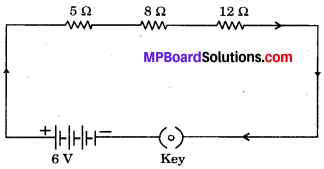Question 2.
Redraw the circuit of question 1, putting in an ammeter to measure the current through the resistors and a voltmeter to measure the potential difference across the 12 Ω resistor. What would be the readings in the ammeter and the voltmeter?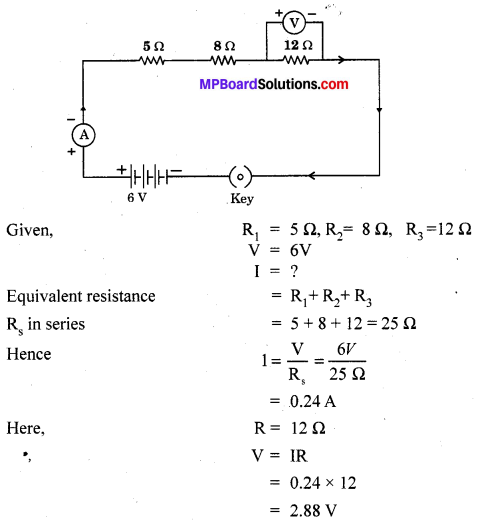Hence, reading in the ammeter would be 0.24 A and reading in voltmeter would be 2.88 V.Class 10th Science Chapter 12 Intext Questions Page No. 216

Question 1.
Judge the equivalent resistance when the following are connected in parallel:
(a) 1Ω, and 106 Ω
(b) 1 Ω, and 103 Ω and 106 Ω
(a) 1 ohm and 106 times ohm
R1 = 1 ohm
R2 = 106 times = 1000000 ohm
Total resistance (parallel)
1/R = 1/R1 + 1/R2
= 1/1 + 1/1000000
= 1000000 + 1 /1000000
= 1000000/ 1000000
1/R = 1/1 ohm (approx.)
∴ Resistance = 1 ohm (approx.)

(b) Given R1 = 1 ohm
R2 = 103 ohm
R3 = 106 ohm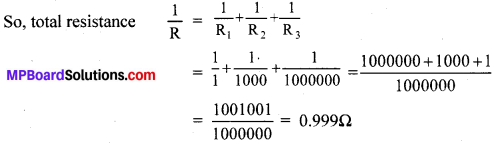Question 2.
An electric lamp of 100Ω, a toaster of resistance 50Ω and a water filter of resistance 500Ω are connected in parallel to a 220 V source. What is the resistance of an electric iron connected to the same source that takes as much current as all three appliances, and what is the current through it?
Resistance of Electric 1 amp R1 = 100Ω
Resistance of Tosser, R2 = 50Ω
Resistance of water filter, R3 = 50Ω
Potential difference, V = 220V
When these are connected in parallel,7.04A of electricity is obtained by three appliances
Resistance of an electric iron connected to the same source that takes as much current as all three appliances∴ Resistance of iron box = 31.25Ω
Electricity flowing through this = 7.04A.

Question 3.
What are the advantages of connecting electrical devices in parallel with the battery instead of connecting them in series?
(i) In parallel circuit, if one electrical appliance stops working due to some defect, then all other appliances keep working normally. In series circuit, if one electrical appliance stops working due to some defect, then all other appliances also stop working.

(ii) In parallel circuits, each electrical appliance gets the same voltage as that of the power supply line. In series circuit, appliances do not get the same voltage, as that of the power supply line.

(iii) In the parallel connection of electrical appliances, the overall resistance of the household circuit is reduced due to which the current from the power supply is high. In the series connection, the overall resistance of the circuit increases too much due to which the current from the power supply is low.

Question 4.
How can three resistors of resistances 2 Ω, 3 Ω, and 6 Ω be connected to give a total resistance of (a) 4Ω, (b) 1Ω?
Given,
R1 = 2 Ω
R2 = 3 Ω
R3 = 6 Ω
(a) When R2 and R3 are connected in parallel with R1 in series we receive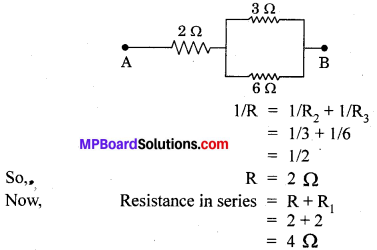(b) When R1, R2, R3 are connected in parallel we receive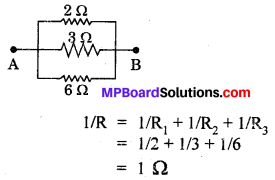Question 5.
What is (a) the highest, (b) the lowest total resistance that can be secured by combinations of four coils of resistance 4 Ω, 8 Ω, 12 Ω, 24 Ω?
(a) For highest resistance according to question resistances must be connected in series: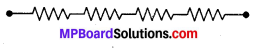4 Ω, 8 Ω, 12 Ω, 24 Ω
R1 = 4 Ω
R2 = 8 Ω
R3 = 12 Ω
R4 = 24 Ω
Total resistance in series = R1 + R2 + R3 + R4
= 4 + 8 + 12 + 24
= 48 Ω
Now, resistance is maximum when connected in series.

(b) For lowest resistance, resistances must be connected in parallel.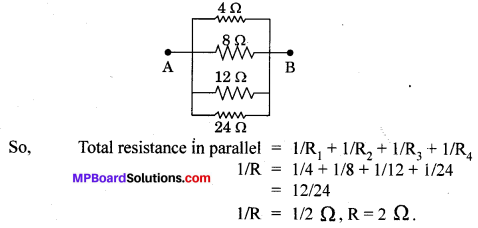Resistance is lowest when connected in parallel.Class 10th Science Chapter 12 Intext Questions Page No. 218

Question 1.
Why does the cord of an electric heater not glow while the heating element does?
In cord of an electric heater, as current flows these become hot arid glows but in case of electric heater this will not happen.

Question 2.
Compute the heat generated while transferring 96000 coulomb of charge in one hour through a potential difference of 50 V.
Given, Charge = 96000 coulomb
Time = 1 hour = 3600 seconds
Potential difference = 50 V Ω
As we know, I = Q/T
I = 96000/36000 = 80/3 amp.
V = I × R
50 = 80/3 × R
So, R = 15/8 Ω
Now, Heat = I2RT
= (80/3)2 × 15/8 × 3600
800 × 6000
= 4800000 joules or 4.8 × 106 J

Question 3.
An electric iron of resistance 20Ω takes a current of 5 A. Calculate the heat developed in 30 s.
As per Joule’s law
H = VIt
H = IR = 5A × 20Ω = 100V
I = 5A, t = 30 sec.
∴ H = 100 × 5 × 30 J
= 15000 J = 1.5 × 104 J.

Class 10th Science Chapter 12 Intext Questions Page No. 220

Question 1.
What determines the rate at which energy is delivered by a current?
The rate at which electric energy is dissipated or consumed in an electric circuit is termed as electric power.
P = VI.

Question 2.
An electric motor takes 5 A from a 220 V line. Determine the power of the motor and the energy consumed in 2 h.
P = VI
V = 220V, and I = 5A.
Power P = 220 × 5 = 1100 W
Power of the motor = P × t
P = 1100 W.
t = 2 Hr 2 × 60 × 60 W
= 7200 S
∴ Energy consumed, E = 1100 × 7200 J
= 7920000
= 7.92 × 106J.

### MP Board Class 10th Science Chapter 12 NCERT Textbook Exercises

Question 1.
A piece of wire of resistance R is cut into five equal parts. These parts are then connected in parallel. If the equivalent resistance of this combination is R’, then the ratio $$\frac { R }{ R` }$$ is –
(a) $$\frac { 1 }{ 25 }$$
(b) $$\frac { 1 }{ 5 }$$
(c) 5
(d) 25
(d) 25

Question 2.
Which of the following terms does not represent electrical power in a circuit?
(a) I2R
(b) IR2
(c) VI
(d) $$\frac { { V }^{ 2 } }{ R }$$
(b) IR2

Question 3.
An electric bulb is rated 220 V and 100 W. When it is operated on 110 V, the power consumed will be –
(a) 100 W
(b) 75 W
(c) 50 W
(d) 25 W
(d) 25 W

Question 4.
Two conducting wires of the same material and of equal lengths and equal diameters are first connected in series and then parallel in a circuit across the same potential difference. The ratio of heat produced in series and parallel combinations would be-
(a) 1 : 2
(b) 2 : 1
(c) 1 : 4
(d) 4 : 1
(c) 1 : 4

Question 5.
How is a voltmeter connected in the circuit to measure the potential difference between two points?
Voltmeter is connected in parallel in the circuit to measure the potential difference between two points.

Question 6.
A copper wire has diameter 0.5 mm and resistivity of 1.6 × 10-8 Ω m. What will be the length of this wire to make its resistance 10 Ω? How much does the resistance change if the diameter is doubled?
Given, Diameter = 0.5 mm = 0.0005 m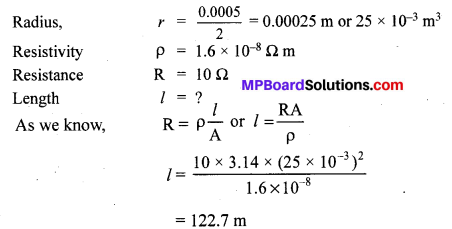So, the resistance is one-fourth if the diameter is doubled.

Question 7.
The values of current I flowing in a given resistor for the corresponding values of potential difference V across the resistors are given below: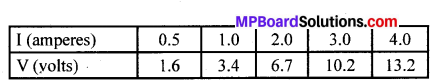Plot a graph between V and I and calculate the resistance of that resistor are given below: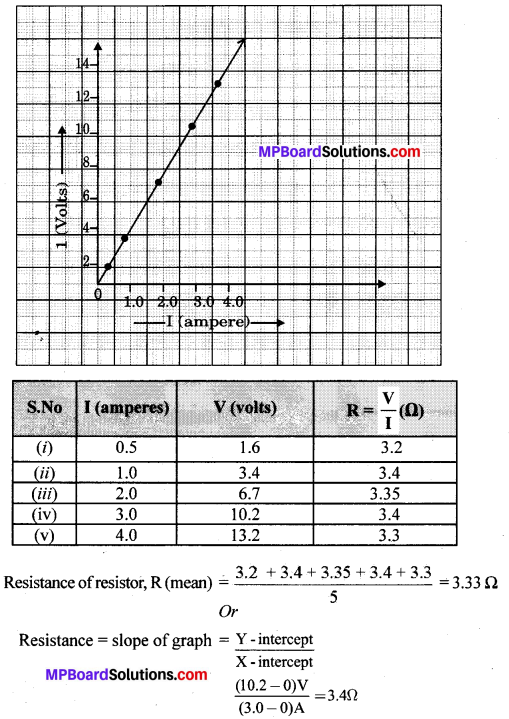Question 8.
When a 12 V battery is connected across an unknown resistor, there is a current of 2.5 mA in the circuit. Find the value of the resistance of the resistor.
Given, V = 12v
I = 2.5 mA = 2.5 × 10-3 A
As we know, R = $$\frac { V }{ 1 }$$
R = $$\frac{12 \mathrm{V}}{2.5 \times 10^{-3} \mathrm{A}}$$ = 48000 Ω
So, R = 4.8 kΩ

Question 9.
A battery of 9 V is connected in series with resistors of 0.2 Ω, 0.3 Ω, 0.4 Ω, 0.5 Ω, and 12 Ω respectively. How much current would flow through the 12 Ω resistor.
R1 = 0.2 Ω R2 = 0.3 Ω R3 = 0.4 Ω R4 = 0.5 Ω R5 = 12 Ω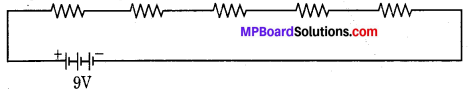Given, V = 9 V
R5 = 0.2 Ω + 0.3 Ω + 0.4 Ω + 0.5 Ω + 12 Ω
= 13.4 Ω
According to formula I = $$\frac { V }{ R }$$
= $$\frac{9 \mathrm{V}}{13.4 \Omega}$$
= 0.67 A
0.67 A current would flow through the 12 Ω resistor.

Question 10.
How many 176 Ω resistors (in parallel) are required to carry 5 A on a 220 V line?
Given
I = 5 A V = 220 V
Now, Let the number of 176 Ω resistors be n.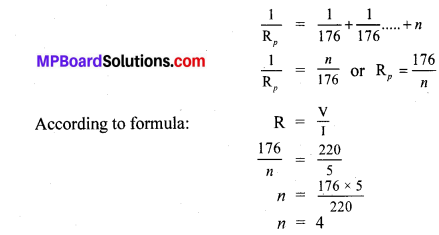So, four resistors of 176 Ω are required to carry 5 A on a 220 V line.

Question 11.
Show how you would connect three resistors, each of resistance 6Ω, so that the combination has a resistance of (i) 9Ω, (ii) 4Ω.
If resistors are connected in series 6Ω + 6Ω + 6Ω =18Ω This ia not correct
When they are connected in parallel
$$\frac{1}{6}+\frac{1}{6}+\frac{1}{6}=3$$ This is also wrong,
i) When they are connected in parallelTwo 6Ω resistors are connected in parallelIf 3rd resistor of 6Ω and 3Ω are connected in series, it becomes 6Ω + 3Ω = 9Ω.
ii) When they are connected in seriesResistance = 6Ω + 6Ω
= 12Ω
If 3rd resistor 6Ω is connected to 12Ω in parallelTotal resistance = 4Ω.

Question 12.
Several electric bulbs designed to be used on a 220 V electric supply line, are rated 10 W. How many lamps can be connected in parallel with each other across the two wires of 220 V line if the maximum allowable current is 5 A?
Here V = 220V
I – 5A∴ 110 lamps can be connected in parallel with each other across the two wires.

Question 13.
A hot plate of an electric oven connected to a 220 V line has two resistance coils A and B, each of 24Ω resistance, which may be used separately, in series, or in parallel. What are the currents in the three cases?
(i) If coils are connected separately V = 220 V
Resistance R1 = 240
As per ohm’s law, V = IR∴ If coils are connected separately 9.16A electricity flows in the coil.
(ii) If coils are connected in series
Resistance R2 = 24Ω + 24Ω = 48Ω
As per ohm’s law V = IR∴ If coils are connected in series 4.58A electricity flows.

Question 14.
Compare the power used in the 20 resistors in each of the following circuits:
(i) a 6 V battery in series with 1Ω and 2Ω resistors, and
(ii) a 4 V battery in parallel with 12Ω and 2Ω resistors.
(i) Potential Difference V = 6V
If 1Ω and 2Ω resistors are connected in series, then Resistance
R = 1 + 2 = 3W.
As per ohm’s law
$$\mathrm{I}=\frac{6}{3}=2 \mathrm{A}$$
P(I2)R = (2)2 × 2 = 8W.
(ii) Potential difference V = 4V
If 12Ω and 2Ω resistors are connected in parallel, voltage is equal
Voltage of resistance 2 W is 4 volts∴ Power of 2Ω is 8 W.

Question 15.
Two lamps, one rated 100 W at 220 V, and the other 60 W at 220 V, are connected in parallel to electric mains supply. What current is drawn from the line if the supply voltage is 220 V?
Both lamps are connected in parallel potential difference = 220 V
Power = V × I.Question 16.
Which uses more energy, a 250 W TV set in 1 hr, or a 1200 W toaster in 10 minutes?
250W TV set in used in 1 Hr, then its energy
= 250 × 3600 = 9 × 105
energy of Toaster = 1200 × 600
If it is used in 10 minutes, then its power = 1200 × 600 × 7.2 × 105 J
∴ Energy of 250 W TV set is used in 1 Hr is greater than 1200 W toaster used in 10 minutes.

Question 17.
An electric heater of resistance 8Ω draws 15 A from the service mains 2 hours.
Calculate the rate at which heat is developed in the heater.
P = I2R
R = 8Ω, I = 15A
P= (15)2 × 8 = 1800 J/s.
∴ Rate at which heat is developed in the heater
= 1800 J/s.

Question 18.
Explain the following:
(a) Why is the tungsten used almost exclusively for filament of electric lamps?
(b) Why arc the conductors of electric heating devices, such as bread-toasters and electric irons, majle of an alloy rather than a pure metal?
(c) Why is the series arrangement not used for domestic circuits?
(d) How does the resistance of a wire vary with its area of cross-section?
(e) Why are copper and aluminium wires usually employed for electricity transmission?
(a) Tungsten used almost exclusively for filament of electric lamps because its melting point is very high and resistivity of tungsten is low, it has special property of glowing on heating, it does not oxidized.

(b) The conductors of electric heating devices such as bread toasters and electric irons are made of alloy because resistivity of an alloy is higher than metal so alloy produces large amount of heat. They do not get oxidized. Melting point is also high.

(c) As in series, circuit voltage is divided. Components of a series circuit receives only small voltage so the amount of current decreases and the device turn hot and do not work properly. Hence, series arrangement is avoided being used in domestic circuits.

(d) Resistance (R) of a wire is inversely proportional to its area of cross-section (A) i.e., when area of cross section decreases the resistance increases or vice versa.

(e) Copper and aluminium are usually used for electricity transmission because they are good conductors of electricity and also have low resistivity and do not get heated.### MP Board Class 10th Science Chapter 12 Additional Important Questions

MP Board Class 10th Science Chapter 12 Multiple Choice Questions

Question 1.
SI unit of an electric current is equal to
(a) 6 × 108 C
(b) 9.8 × 1010 C
(c) 1.6 × 10-19 C
(d) None of these
(d) None of these

Question 2.
Which materials have changing resistivity?
(a) Conductors
(b) Semiconductors
(c) Non-metals
(d) Super metals
(b) Semiconductors

Question 3.
If wire in a circuit is doubled its resistivity will be?
(a) Two times
(b) Ten times
(c) Zero
(d) half
(d) half

Question 4.
A wire used in circuit must be
(a) Highly resistant
(b) Non resistance
(c) Have high melting point
(d) None of these
(b) Non resistance

Question 5.
A heating w ire must have
(a) High melting point
(b) High conductivity to heat
(c) Both
(d) None
(b) High conductivity to heat

Question 6.
SI unit of resistance is:
(a) Coulomb
(b) Ampere
(c) Ohm
(d) Ohm – meter
(c) Ohm

Question 7.
Symbol for use of voltmeter in circuit is: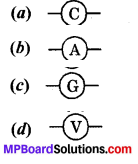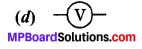Question 8.
Symbol for use of battery in circuit is: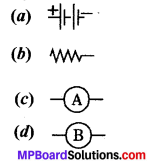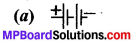Question 9.
Relation of potential difference and current is represented by:
(a) Joule’s law
(b) Ohm’s law
(c) Dalton’s law
(d) None
(b) Ohm’s law

Question 10.
Formula which represents the relation among potential difference and current following in a circuit is:
(a) V = IR
(b) V = I/R
(c) l = Q/t
(d) R = p $$\frac { l }{ A }$$
(a) V = IR

Question 11.
Kilo watt is a unit for:
(a) Power
(b) Electrical energy commercial
(c) Resistance
(d) Current flow in circuit
(a) Power

Question 12.
Which one has less resistance?
(a) 10 watt bulb
(b) 15 watt LED
(c) 60 watt bulb
(d) 60 watt LED.
(a) 10 watt bulb

Question 13.
In which type of combinations resistance is more?
(a) Series Combination
(b) Parallel combination
(c) both
(d) Single resistance unit.
(a) Series Combination

Question 14.
Joule’s law formulation is
(a) H = IRT
(b) H = I2RT
(c) P = VI
(d) RT = PV
(d) RT = PV

Question 15.
Hardest metal which can resist maximum heat is:
(b) Tungsten
(c) Silver
(d) Carbon
(b) Tungsten

Question 16.
If current passing a circuit is 10 A and wire is connected to 100 volt line resistant in the wire will be:
(a) 10 A
(b) 10 Ω
(c) 100 Ω
(d) O Ω
(b) 10 Ω

Question 17.
An insulator has a resistivity equals to:
(a) 10-8 Ω m
(b) 10 Ω m
(c) 5 Ω m
(d) 10-18
(a) 10-8 Ω m

Question 18.
Calculate current-(I) in circuit given below:
(a) 0.6 A
(b) 20 A
(c) 50 A
(d) 10 A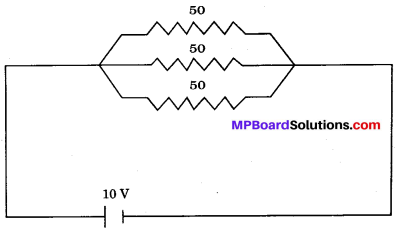(a) 0.6 A

Question 19.
If resistors are arranged in parallel with value 1 Ω, 2 Ω and 3 Ω Total resistant will be:
(a) more than 3 Ω
(b) less than 3 Ω
(c) less than 1 Ω
(d) equal to 1 Ω
(b) less than 3 Ω

Question 20.
The changing 1 kWh to joule will be equal to
(a) 3.6 × 106 J
(b) 3.6 × 10-6 J
(c) 1.6 × 106 J
(d) 1 × 106 J
(a) 3.6 × 106 JMP Board Class 10th Science Chapter 12 Very Short Answer Type Questions

Question 1.
What kind of circuit is required for flow of current ?
Closed.

Question 2.
Draw a schematic diagram of a simple electric with battery, bulb, ammeter and key.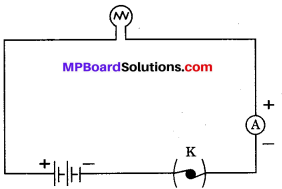Question 3.
Express in formula form:

1. net charge of a circuit
2. time taken for production of a unit amount of current.

1. Q = It.
2. t = Q/I.

Question 4.
What is the value of an electric charge for an electron?
-1.6 × 10-19 C.

Question 5.
Formulate relationship between potential difference and current.
V = IR.

Question 6.
Draw the graph verifying ohm’s law.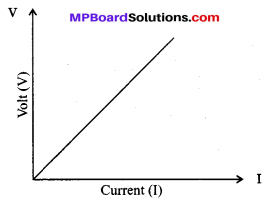Question 7
Draw circuit with three resistor in series combination.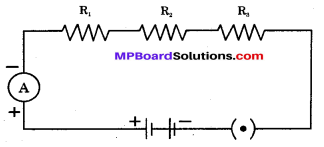Question 8.
Represent with diagram:
(i) A cell
(ii) A bulb.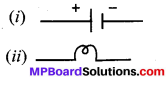Question 9.
Which material has highest conductivity and least resistance?
Super conductors.

Question 10.
Which quality of electrical energy is inversely proportional to electrical power?
Resistance.

Question 11.
Calculate resistance between terminal P & Q of following diagram.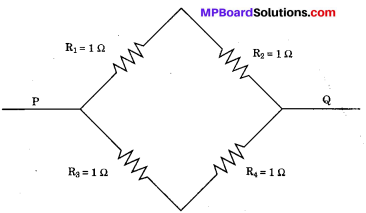First R1 and R2 are in series.
So, R’ = R1 + R2 = 1 Ω + 1 Ω = 2 Ω.
then, R3 and R4 are in series
R” = R3 + R4 = 1 Ω + 1 Ω = 2 Ω
Now both R’ and R” are in parallel.
$$\frac { 1 }{ R }$$ = $$\frac{1}{R^{\prime}}+\frac{1}{R^{\prime \prime}}+\frac{1}{2}+\frac{1}{2}=1$$
So, R = 1 Ω

Question 12.
If a circuit has open key will current flow from it?
No.

Question 13.
If ammeter is not placed in circuit will current flow?
Yes.

Question 14.
Which gas is filled in electric bulb?
Argon.

Question 15.
Write the formula representing relationship between heat produced and current flowing in a circuit.
H = I2RT.

Question 16.
Write the formula to calculate power generated by a circuit a with current M.
Since, P = VI
Putting value of I, P = VM (I = m, Given)

Question 17.
Convert 5 kWh in joule.
1 kWh = 3.6 × 106 Joule
5 kWh = 5 × 3.6 × 106 J.
= 16 × 106 J
or 1.6 × 107 J

Question 18
One volt ampere is equal to how many watt power?
1 Watt Power.MP Board Class 10th Science Chapter 12 Short Answer Type Questions

Question 1.
What is electric current?
Total charge which pass through a particular area in unit time is called electric current of that particular conductor.

Question 2.
What is potential difference?
Movement of electron need a pressure difference among conductors. Hence, battery or current flow suppliers are added to circuit. This difference in potential is called potential difference.

Question 3.
Define Ohm’s law.
Ohm’s law states that current flowing in a circuit is directly proportional to its potential difference. It is represented by formula,
V α I
or V = RI
or V = IR
Here, R is a constant known as resistance.

Question 4.
Why resistance is applied to a circuit?
To control the current flow in desired way resistance is applied. Example- Regulator of fan give desired speed of wind flow by controlling flow of current which is being converted to physical energy by fan.

Question 5.
Write down the factors at which the resistance of the conductor depends.

1. Length of wire: Resistance is directly proportional to length R ∝ L.
2. Area cross section: Resistance is inversely proportional to area cross section of a wire R ∝ 1/A.
3. Nature of material.
4. Temperature of the conductor.

Question 6.
Arrange the resistivity in decreasing order of following material,
Iron, Silver, Tungsten, Manganin and Glass
Glass > Manganin > Iron > Tungsten > Silver.

Question 7.
Give reason for the following:

1. Electric bulbs are usually filled with chemically inactive gases like nitrogen and argon.
2. Copper and aluminium wires are usually employed for electricity transmission.
3. Fuse wire is placed in series with the device.

1. Electric bulbs are usually filled with chemically inactive gases like nitrogen and argon because these gases do not react with the hot tungsten filament and hence, prolong the life of filament of the electric bulb.
2. Copper and aluminium wires are usually employed for electricity transmission because copper and aluminium have low resistivity and thus, they are very good conductors of electricity.
3. Fuse wire is placed in series with the device because when large current passes through the circuit the fuse wire gets heated up and melts and whole circuit breaks and the device is protected from the damage.

Question 8.
If R1 = 10 Ω, R2 = 20 Ω and R3 = 30 Ω, calculate the effective resistance when they are connected in series to a battery of 6 V. Also find the current flowing in the circuit.
(a) Current flows through different resistances, when these are joined in series, as shown in the below figure: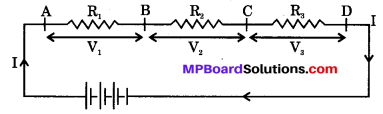(b) R1 = 10 Ω, R2 = 20 Ω, R3 = 30 Ω
Effective resistance, R = R1 + R2 + R3
R 10 + 20 + 30 = 60 Ω
Potential difference, V = 6V,
Current, I = ?
According to Ohm’s law
V = IR

Question 9.
Find the resistance between points A and B in the circuit diagram given below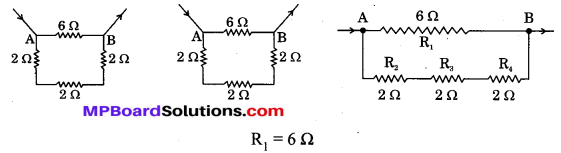R2, R3, R4 are in series and have resultant resistance R
R’ = R2 + R3 + R4
= 2 + 2 + 2 = 6 Ω
R’ is in parallel combination with R1
∴ Resultant resistance of the circuit (R’)
⇒ $$\frac { 1 }{ R }$$ = $$\frac{1}{R_{1}}+\frac{1}{R_{1}}$$ = $$\frac { 1 }{ 6 }$$ + $$\frac { 1 }{ 6 }$$ = $$\frac { 2 }{ 6 }$$ = $$\frac { 1 }{ 3 }$$
∴ Resistance, R = 3 Ω.MP Board Class 10th Science Chapter 12 Long Answer Type Questions

Question 1.
An electric iron consumes energy at a rate of 840 W when heating is at the maximum and 360 W when the heating is at the minimum. The voltage at which it is running is 220 V. What are the current and resistance in each case?
Solution:
At maximum heating:
The consumption of energy (electric) is given at the rate of 840 W at voltage 220 V.
P = 840 Ω, V = 220 V
Then current, I1 = ?
∴ P = V × I1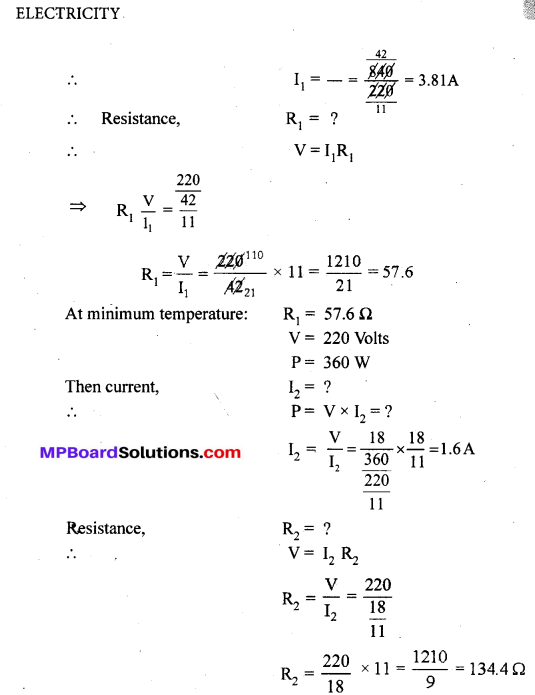Question 2.
Three resistors of 5 Ω,10 Ω and 15 Ω are connected in series and the combination is connected to battery of 30 V. Ammeter and Voltmeter are connected in the circuit. Draw a circuit diagram to connect devices all the in correct order. What is the current flowing and potential difference across 10 Ω resistance?
Current flowing I = ?
V2 = ?
Total resistance, R = R1 + R2 + R3
= 5 + 10 + 15 = 30 Ω
Total potential difference, V = 30 volts
According to Ohm’s law V = IR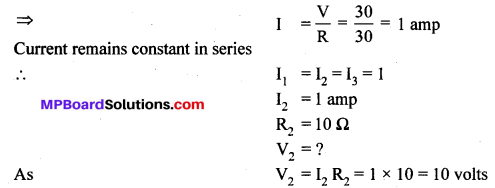Potential difference across 10 Ω is 10 volts.

Question 3.
Nichrome wire of length ‘l’ and radius ‘r’ has resistance of 10 Ω. How would the resistance of the wire change when:
(a) (i) Only length of the wire is doubled?
(b) Why element of electrical heating devices are made up of alloys?
Solution:
(a) Resistance, R ∝ 1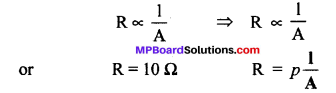(i) Resistance is directly proportional to the length of the conductor. If length of nichrome wire (I) is doubled its resistance also gets doubled.
∴ R’ new resistance = 20 Ω

(ii) The resistance of the wire is inversely proportional to the square of its diameter. If the diameter of the wire is doubled, its resistance becomes one-fourth.
∴ R’, new resistance = $$\frac { 10 }{ 4 }$$ Ω = 2.5 Ω.

(b) The heating elements of electrical heating appliances are made up of nichrome alloy because,

• Nichrome has very high resistivity due to which it produces a lot of heat on passing current.
• Nichrome does not undergo oxidation easily even at high temperature, it can be kept red hot without burning.

Question 4.
The resistance of a wire of 0.01 cm radius is 10 Ω. If the resistivity of the material of the wire is 50 × 10-8 ohm meter, find the length of the wire.
Resistance of a wire, R = 10 Ω Radius, r = 0.01 × 10-2 m
Resistivity, p = 50 × 10-8 ohm meter Length of the wire, l = ?
Area of cross section, A = πr2 = 3.14 × (0.01 × 1o-2)2 m2
= 3.14 × 0.01 × 0.01 × 10-4 m2
= 3.14 × 10-8 m2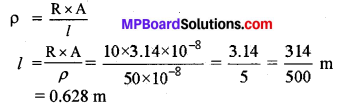Question 5.
(a) Calculate the resistance of the wire using the graph.
(b) How many 176 Ω resistors in parallel are required to carry 5 A on a 220 V line?
(c) Define electric power. Derive relation between power, potential difference and resistance.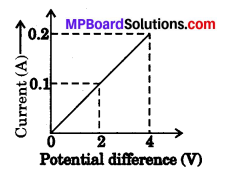(a) Resistance of wire = Slope of the graph
According to Ohm’s law,
V = IR or R = $$\frac { V }{ I }$$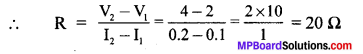(b) Resistance, R1 = 176 Ω, No. of resistors = n,
Current, I = 5A
Potential difference, V = 220 volts, Resultant resistance = R According to Ohm s law,
V = IR, R = $$\frac { V }{ 1 }$$ = $$\frac { 220 }{ 5 }$$ = 44 Ω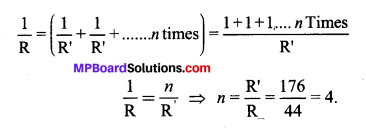Thus, 4 resistors of 176 Ω in parallel combination are required to carry 5 A on a 220 V line.

(c) Electric power is defined as the electrical work done per unit-time.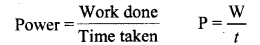The work done, W by current, I when it flows for time t under potential difference V is given by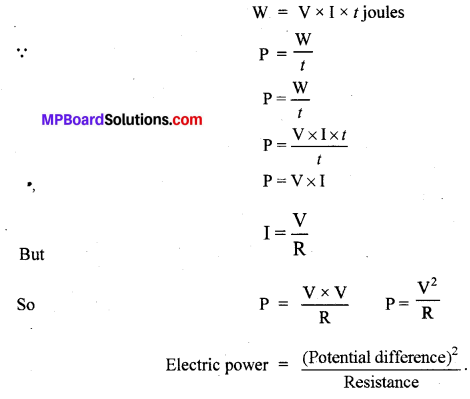### MP Board Class 10th Science Chapter 12 NCERT Textbook Activities

Class 10 Science Activity 12.1 Pages No. 203.204

• Set up circuit as shown in figure, consisting of a mchrome wire XY of length, say 0.5 m, an ammeter, a voltmeter and four cells of 1.5 V each (Nichrome is an alloy of nickel, chromium, manganese, and iron metals.)
• First use only cell as the source in the circuit. Note the reading of the voltmeter V for the potential difference across the nichrome wire XY in the circuit. Tabulate them in table given.
• Next connect two cells in the circuit and note the respective readings of the ammeter and voltmeter for the values of current through the nichrome wire and potential difference across the nichrome wire.
• Repeat the above steps using three cells and then four cells in the circuit separately.
• Calculate the ratio of V to 1 for each pair of potential difference V and current I.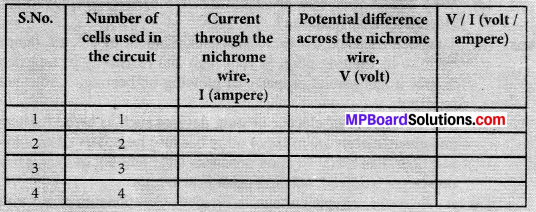• Plot a graph between V and 1, and observe the nature of the graph.

Observations: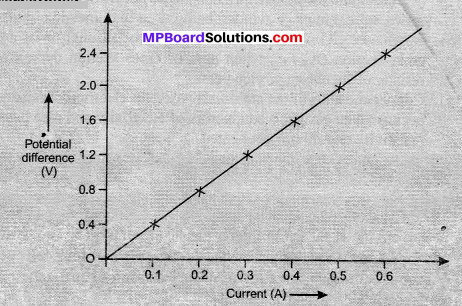Approximately same value for $$\frac { V }{ I }$$ will be obtained in each case. The straight line is obtained in the V – I graph. Thus, $$\frac { V }{ I }$$ is a constant ratio.

Class 10 Science Activity 12.2 Page No. 205

• Take a nichrome wire, a torch bulb, a 10 W bulb and an ammeter (0-5 A range), a plug key and some connecting wires.
• Set up the circuit by connecting four dry cells of 1.5 V each in series with the ammeter leaving a gap XY in the circuit as shown in Fig. 12.1.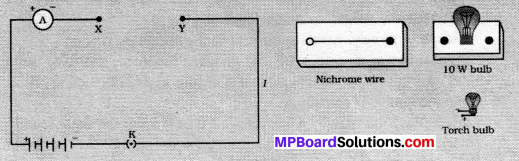• Complete the circuit by connecting the nichrome wire in the gap XY. Plug the key. Note down the ammeter reading. Take out the key from the plug. [Note: Always take out the key from the plug after measuring the current the circuit.
• Replace the nichrome wire with the torch bulb in the circuit and find the current through it by measuring the reading of the ammeter.
• Now repeat the above step with the 10 W bulb in the gap XY.
• Are the ammeter readings differ for different components in the gap XY? What do the above observations indicate?
• You may repeat this Activity by keeping any material component in the gap. Observe the ammeter readings in each case. Analyse the observations.

Observations:

• The current is different for different components as certain components offer an easy path for the flow of electric current while the others resist the flow.

Class 10 Science Activity 12.3 Page No. 206

• Complete an electric circuit consisting of cell, an ammeter, a nichrome wire of length l [say, marked (1)] ana a plug key, as shown in Fig. 12.2.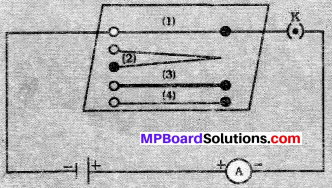Electric circuit to study the factors on which the resistance of conducting wires depends.
• Figure 12.2. Electric circuit ti study the factors on which the resistance of conducting wires depends
• Now, plug the key. Note the current in the ammeter.
• Replace the nichrome wire by another nichrome wire of same thickness but twice the length, that is 2l [marked (2) in the Fig. 12.2],
• Now replace the wire by a thicker nichrome wire of the same length / [marked (3)]. A thicker wire has a larger cross-sectional area. Again note down the current through the circuit.
• Instead of taking a nichrome wire, connect a copper wire [marked (4) in Fig. 12.2] in the circuit. Let the w ire be of the same length and same area of cross-section as that of the first nichrome wire [marked (1)]. Note the value of the current.
• Notice the difference in the current in all cases.
• Does the current depend in the length of the conductor?
• Does the current depend on the area of cross-section of the wire used?

Observations:

• The length of the conductor affects the current flow. Ammeter reading decreases to one-half when the length of the wire is doubled.
• The cross-section of the wire also affects the current flow. The length and cross-section changes affect the resistance also.

Class 10 Science Activity 12.4 Page No. 210

• Join three resistors of different values in series. Connect them with a battery, an ammeter and a plug key. as shown in Fig. 12.3. You may use the resistors of values like 1Ω, 2Ω, 3Ω etc, and a battery of 6 V for performing this Activity.
• Plug the key. Note the ammeter reading.
• Change the position if ammeter to anywhere in between the resistors. Note the ammeter reading each time.
• Do you find any change in the value of current through the ammeter?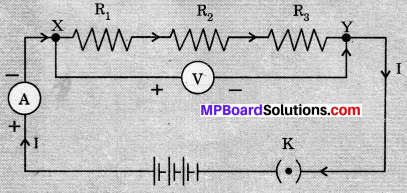Observations:

• The value of the current in the ammeter remains unchanged. This shows that it is independent of its position in the electric current. This shows that the current is the same in every part of a series combination.

Class 10 Science Activity 12.5 Page No. 211

• In Activity 12.4, insert a voltmeter across the ands X and Y of the series combination of three resistors, as shown in Fig. 12.4.
• Plug the key in the circuit and note series combination of resistors. Let it be V. Now measure the potential difference across the two terminals of the battery. Compare the two values.
• Take out the plug key and disconnect the voltmeter. Now insert the voltmeter across the ands X and P the first resistor, as shown in Fig. 12.4.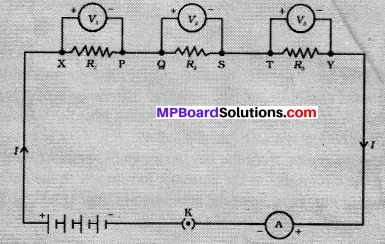• Plug the key and measure the potential difference across the first resistor. Lei it be V1.
• Similarly, measure the potential difference across the first resistor, separately. Let these values be V3, and V2, respectively.
• Deduce a relationship between V, V1, V2 and V3.

Observations:

• The potential difference (V) is equal to the sum of potential differences V1, V2, and V3.
• Total potential difference across a combination of resistors in series is equal to the sum of potential difference across the individual resistors,
V = V1 + V2 + V3

Class 10 Science Activity 12.6 Page No. 211

• Make a parallel combination. X Y of three resistors having resistances R1, R2, and R3, respectively Connect it with a battery, a plug key and an ammeter, as shown in Fig. 12.5. Also connect a voltmeter in parallel with the combination of resistors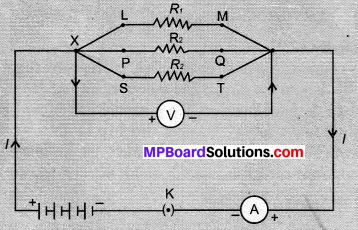• Plug the key and note the ammeter reading. Let the current be I. Also take the voltmeter reading. It gives the potential difference V, across the combination. The potential difference across each resistor is also V. This can be checked by connecting the voltmeter across each individual resistor (see Fig. 12.6).
• Take out the plug from the key. Remove the ammeter and voltmeter from the circuit. Insert the ammeter in series with the resistor Rl, as shown in Fig. 12.6. Note the ammeter reading, 11.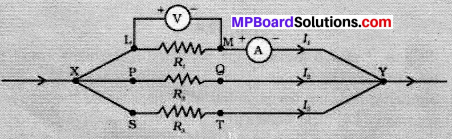Similarly, measure the currents through R2 and R3 Let these be I2, and I3, respectively. What is the relationship between I, I1, I2 and I3?

Observations:

• The total current I, is equal to the sum of the separate currents through each branch of the combination,
I = I1 + I2 + I3